# Class 12 Economics Chapter 4 Income Determination

Class 12 Economics Chapter 4 Income Determination Question answer to each chapter is provided in the list so that you can easily browse through different chapters HS 2nd Year Economics Notes, AHSEC Class 12 Economics Chapter 4 Income Determination, Class 12 Economics Question Answer In English Notes and select needs one.

## Class 12 Economics Chapter 4 Income Determination

Also, you can read the NCERT book Notes Class 12 Economics Chapter 4 Income Determination online in these sections Solutions by Expert Teachers as per SCERT Class 12 Economics Chapter 4 Income Determination (CBSE) Book guidelines. These solutions are part of AHSEC All Subject Solutions. Here we have given Assam Board Class 12 Economics Chapter 4 Income Determination Solutions for All Subjects, You can practice these here in Class 12 Economics Chapter 4 Income Determination.

### Income Determination

Chapter: 4

PART – A

1. What is aggregate demand?

Ans : Aggregate demand refers to the total expenditure that the residents of a country are willing to spend on the purchase of goods and service during a year.

2. Given the marginal propensity to save to be 0.3, find out the income multiplier.

Ans : Given, MPS = 0.3

3. What is the literacy meaning of ‘ceteris paribus’?

Ans : Other things remaining constant.

4. Write true or false:

In an equilibrium state of the economy, when the final goods market is in equilibrium, the ex-ante supply is equal to the ex-ante demand.

Ans : True

5. Fill in the blanks:

The value of planned consumption is called consumption. (ex-ante/ ex-post).

Ans : Ex-ante.

6. Fill in the blanks:

The planned value of a variable as opposed to its actual value is called (Ex-post/ex-ante/Inventory).

Ans : Inventory.

7. Name the situation is where people become more thrifty and they end up saving less or same as before in aggregate.

Ans : Propensity to save.

8. Choose the correct one:

Shift of a graph due to change in the value of a parameter is called (graphic shift/ parametric shift).

Ans : Parametric shift.

9. Choose the correct one:

The ratio of additional consumption to additional income is called (marginal propensity to same/ marginal propensity to consume/ marginal income)

Ans : Marginal propensity to consume (MPC)

10. Show the relationship between multiplier and marginal propensity to consume.

Ans : k = 1/1-MPC

11. State any two measures of fiscal policy to correct the problem of excess demand in an economy.

Ans : (a) To control the situation of excess demand, Government should reduce its expenditure to the maximum possible extent.

(b) More emphasis should be placed to reduce expenditure on defence and unproductive works as they rarely help in growth of a country.

12. What is the difference between Ex-ante investment and Ex-post investment?

Ans : Ex-ante investment refer to the planned investment or desired investment.

Ex-post investment refer to actual investment in the economy.

B. SHORT ANSWER QUESTION TYPE –II: (MARKS: 3)

1. What is marginal propensity to save>

Ans : It is the ratio of change in savings to change in income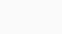2. Why do we assume that the prices vary only in the long-run?

Ans : Because in the long run both demand and supply can change in the market.

3. What is output multiplier?

Ans : Output multiplier is related with the value of Marginal propensity consume (MPC). It is expressed as, Output multiplier = 1/1-MPC

4. What is consumption expenditure?

Ans : It implies to the total expenditure incurred by households on the purchase of goods and services during a particular period, i.e., one year.

5. If marginal propensity to consume (c) of an economy is 0.9, find out the value of income multiplier.

Ans : Given, MPC = 0.9

6. The autonomous consumption of an economy is Rs. 500 crores, autonomous investment is Rs. 150, government expenditure is Rs. 200 crores and personal disposable income is Rs. 600 crores. If the marginal propensity to spend is 0.8, find out the aggregate level of income of that economy.

Ans : We know that,

Y = C+I+G= (a+by) + I+G = (500+0.8 x 600) + 150+200=850+480= 133cr.

7. What is paradox of thrift – explain.

Ans : The paradox of thrift has been popularised by John Maynard Keytnes. This concept states that if everyone tries to save an increasingly larger proportion of income, they would become poorer instead of richer. This is because the economy will slow down from the reduction in demand and the very same people will loose their jobs. This theory however applies mainly to Keynesian economics where increased saving represents a diminishing circular flow of income.

8. What consists of aggregate demand for final goods?

Ans : Aggregate demand for final goods consist of consumption demand(c) investment demand (i), Government’s demand for goods and services (G), Net demand by foreigners, i.e. net exports (X – M). i.e AD = C + I + G + X – M

C implies demand for goods and services by the households of a country in a year.

I refers to the planned or ex-ante investment expenditure by the private firms.

G includes both government consumption expenditure and Government investment expenditure.

X – M is the net export.

9. Show that the output multiplier is equal to zero where C = MPC.

Ans : Higher MPC implies more consumption expenditure and vice versa. As a result of this increase in consumption expenditure, there will be excess demand in the economy. To meet this increased demand, producers will have to rundown their inventory. In the next production cycle, they will plan to increase their output to restore equilibrium. The extra output so produced is distributed among various factors of production as factor payments. Hence the income of the economy goes up by this extra output produced to meet extra demand. When income increases as a result of extra output, consumption expenditure again goes up. It will increase by MPC x∆y since people spend a fraction (= MPC) of their additional income on consumption. Hence in the next round, aggregate demand in economy again goes up by further to restore equilibrium. Like before, this extra output will generate extra factor income in the economy. This process goes round after round, with producers increasing their output to meet the excess demand in each round and consumers spending a part of their additional income this extra production or income on consumption there by creating further excess demand is the next round.

C. SHORT ANSWER QUESTION TYPE – I : (MARKS: 4)

1. The level of autonomous consumption of an individual is Rs. 500 and his personal disposable income is Rs. 500. If this marginal propensity to consume is 0.8, find out the level of aggregate consumption.

Ans : We know that,

C = a + by = 500+0.8 x 5000 = 500+4000 = 4500cr.

2. The marginal propensity to consume (c) of an economy is 0.85, find out –

(i) the size of the income multiplier,

(ii) the income t generated in the economy if the government investment in increased by Rs. 250 crores.

3. What is ex ante and ex post?

Ans : Savings, which are planned (intended) to be made by all households in the economy during a period, in the beginning of the period is called planned or ex-ante savings.

The investment, which is planned to be made by all the firms or entrepreneurs in the economy during a period, in the beginning of the period is called ex-ante investment.

Output, is at its equilibrium point, when aggregate quantity demanded = aggregate quantity supplied.

Ex-post saving refers to the actual saving in an economy during a year.

4. Explain the concept of ex ante consumption.

Ans : Ex ante consumption refers to the planned consumption which is mode by all the households in the economy.

5. Distinguish marginal propensity to consume from marginal propensity to save.

Ans : The ratio of change in consumption to change in income is termed as marginal propensity to consume. i.e. =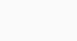It is the ratio of change in savings to change in income6. Explain the concept of ex ante investment.

Ans : Ex-ante investment refer to amount of investment, which firms plan to invest at different levels of income in the economy. The amount of ex-ante or planned investment is determined by the relation between investment demand and rate of interest i.e. by investment demand function.

7. What are the components of aggregate demand? Explain briefly.

Ans : Aggregate demand refers to the planned expenditure of the households on the purchase of goods and services in an accounting year.

Components of aggregate demand (AD) = C+I+G= (X-N)

Where C = household consumption demand

I = Private investment demand

G = government’s demand for goods and services

(X-N) = Net demand by foreigners.

8. What is aggregate supply? What are the components of aggregate supply.

Ans : Aggregate supply means total output of goods and services produced in an economy. Thus we can say that aggregate supply and national income are the same thing. A major part of the national income is spent on consumption and the rest is saved. So, national income is the sum total of AS = C+S where C = Consumption

S = Saving

9. Distinguish between autonomous investment and induced investment.

Ans : Investment which is independent of income level is called autonomous investment.

Investment that depends upon the level of income is known as induced investment.

10. What are the determinants of investment demand?-Explain.

Ans : Investment refers to the expenditure incurred on creation of new capital assets. It includes the expenditure incurred on assets like machinery, building, equipment, raw material, etc. which lead to increase in the productive capacity of an economy.

The investment expenditure is classified under two heads: (i) Induced Investment, (ii) Autonomous Investment

Induced investment refers to the investment, which depends on the profit expectations and is directly influenced by income level and autonomous investment refer to the investment, which is not affected by changes in the level of income and is not induced solely by profit native.

The main determinates of investment are: (i) Marginal efficiency of investment (MEI), (ii) Rate of interest

Marginal efficiency of investment refers to the expected rate of return from an additional investment. MEI is determined by two factors –

(a) Supply price: It refers to the cost of producing a new assets of that kind. It is the price at which, the new capital asset can be supplied or replaced.

(b) Prospective yield: It refers to net return expected from the capital asset over its life time.

Rate of interest refers to cost of borrowing money for financing the investment. There exists an inverse relationship between rate of interest and the volume of investment. At a high rate of investment, the investment spending will be less and vice-versa.

11. What is investment multiplier? Explain with the help of a diagram.

Ans : The concept of multiplier is an important contribution of prof. J.M. Keynes. Keynes believed that an initial increment in investment increases the final income by many times. The literal meaning of multiplier is multiple. In an economy, when there is increase in investment by a certain amount, the resultant change in income is multiple of change in investment. The multiplier is defined as the ratio of the change in income to change in investment.

Algebraically,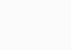Where K is change in income and ∆I is the change in investment

The concept of multiplier is diagrammatically presented with the help of AD and AS approach.

In the diagram, initial equilibrium is determined at point E, where AD curve intersects the AS curve. The equilibrium level of income is OY.

Now, suppose investment increases by ∆I, so that the new aggregate demand curve (AD1) intersects the aggregate supply curve (AS) at point F. The new equilibrium level of income is OY. It is clear from the diagram that the increase in income (YY1 or ∆Y is greater than increase in investment (∆I). The value of multiplier is given by12. Explain the principle of effective demand with the help of diagram.

Ans : The particular level of AD which is equal to AS is called effective demand. The level of effective demand determines the level of income, output and employment. Aggregate effective demand refers to that level of AD, where AD = AS. It correspondence to the equilibrium level of income and output.

In the diagram AD and AS are the aggregate demand and aggregate supply curve respectively. AD function shows various levels of AD like E0 EE1. But it is only at point E the level of AD (= EY) is equal to the level of AS. Hence E point on AD curve represents effective demand for increasing the level of income and output in the economy, aggregate effective demand most increase.

13. Explain the working of the multiplier mechanism in the final goods market with the help of table.

Ans : The multiplier is defined as the ratio of the change in income to the change in investment. The working of the multiplier mechanism is shown with the help of a table below:

The multiplier process (MPC = 0.75)

Suppose there is an initial increase in investment of Rs. 100 crores in the economy. Further suppose the value of MPC is 0.75. Their increase of investment of Rs. 100 crore results an increase of income of Rs. 100 of people working in the investment industry. They will together spend Rs. 75 (3/4 of 100) on consumption goods. Their increase in consumption of Rs. 75 crores will increase the income of people working in consumption goods industry in the second round. This additional income of Rs. 75 crores will lead to an additional consumption of Rs. 56.25 crores in the economy. This is the time increase in the level of income in the economy. Since an additional income of Rs. 56.25 crores has been generated in the economy, it results in increase in consumption by Rs. 42.19 (3/4 of 56.25 crores) This will curve further increase in income in the economy. This process will continue with each round of income being ¾ times the previous level. Thus there are many rounds of increase in income due to increase investment ∆I in the first round ∆y = ∆I but in subsequent rounds ∆Y = ∆C

The table shows that initial increase in investment of Rs. 100 crores has resulted in an increase in income of Rs. 400 crores. This is the resultant increase in income is multiple of initial increase in investment. Thus in this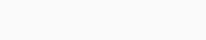14. ” As people become more thrifty they end up saving less or same as before.” Explain.

Ans : “As people become more thrifty they end up saving less or as before” There i a direct relationship between income and savings. As income increases, level of savings in the economy also increases, but by less than increase in income. It means as income increases, proportion of income round increases. At any level of income below the break even incomes savings are negative and at income levels higher than break even income, savings are positive.

15. The autonomous consumption of an individual is Rs. 500 and hi personal disposable income is Rs. 5000. If his marginal propensity to consume is 0.8, find out the level of aggregate consumption.

Ans We are given,

Autonomous consumption = Rs. 500

Disposable income = Rs. 5000

MPC = 0.8

According to formula

We know that consumption function is –

C = a+by here, a = Rs. 500

C = 500 + 0.8×5000 b = MPC = 0.8

= 500 + 8/10 x 500∅ y = Rs. 5000

= 500 + 4000 = 4500

C = 4500

Where, C = Aggregate consumption.

Aggregate consumption = Rs. 4500

16. Measure the level of ex-ante aggregate demand when autonomous investment and consumption expenditure (A) is Rs. 50 crores, MPS is 0.2 and level of income (y) is Rs. 4,000 crore. State whether the economy is in equilibrium or not (cite reasons). 2018

Ans : We know, MPC = 1 – MPS = 1 – 0.2

∴ MPC = 0.8

Again, AD = C + I = c + by + I = A + by [∵A = c + I]

= 50 + (0.8) x 4000 = 50 + 3200 = Rs. 3250 crores

Since AS (y) is Rs. 4000 crores, and

AD (which is Rs. 3250 crores) falls short of AS.

Hence, the economy is not in equilibrium.

D. LONG ANSWER QUESTIONS TYPE: (MARKS: 5)

1. Analyses the concept of ex ante aggregate demand for final goods in an economy without government.

Ans : (i) If planned saving are greater than planned investment, i.e. after point E as shown in figure, it means that households are not consuming as much as the firms expected them to. As a result, the inventory rises above the desired level. To clear the unwanted increase in inventory, firms would plan to reduce the production till savings and investment become equal to each other.

(ii) If planned savings are less than planned investment i.e before point ‘E’ as shown in the above diagram, it means that households are consuming more and savings less than what the firms expected them to. As a result, the planned inventory starts falling and moves below the desired level. To bring the inventory back to the desired level, firms plan to increase the production till saving and investment become equal to each other.

2. Explain the relationship between investment multiplier and marginal propensity to consume.

Ans : A constant final goods price and constant rate of interest over short run determine the level of aggregate demand for final goods in the economy. The aggregate supply is perfectly elastic at this price. Under such circumstances, aggregate output is determined solely by the level of aggregate demand. This is known as effective demand principle.

The increment in equilibrium value of total output thus exceeds the initial increment in autonomous expenditure. The ratio of the total increment in equilibrium value of final goods output to the initial increment in autonomous expenditure is called the output multiplier of the economy.

The output multiplier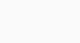where ∆y is the total increment in final goods output and C = MPC. The size of the multiplier depends on the value of C. As c becomes larger, the multiplier increases.

3. Explain the process of equilibrium income determination of an economy with the use of aggregate demand and aggregate supply curves.

Ans : According to the Keynesian theory, the equilibrium level of income in an economy is determined when aggregate demand, represented by C + I curve is equal to the total output (aggregate supply).

Income/Output employment

In the figure, the AD or (C + I) curve shows the desired level of expenditure by consumers and firms corresponding to each level of output. The economy is in equilibrium at point ‘E’ where (C + I) curve intersects the 45° line. OY i the equilibrium level of income. If there is any deviation from the equilibrium level of output, i.e., when planned spending (AD) is not equal to planned output (AS), then a process of readjustment will start in the economy and the output will tend to adjust up or down until AD and AS are equal again.

Scroll to Top